```

```
Wholeness and the Implicate Order
```
```
The Crystallization Process and the Implicate Order
(Part Four)

```

```
We  will now investigate the generation of the Plane Group P2gg, i.e. the generation of its group elements.
```
```
The Plane Group P2gg

The primitive Rectangular Net can also accommodate motifs possessing only 2-fold rotational symmetry, i.e. motifs having a point symmetry 2. Figure 1 shows a regular array of such motifs, based on a primitive rectangular net. The pattern represents the Plane Group P2gg.Figure 1.  Motifs possessing only a 2 symmetry can be accommodated in a primitive 2-D rectangular lattice, resulting in a periodic structure. This structure must be conceived as extending indefinitely in two-dimensional space.

```
```
The total symmetry content of the Plane Group P2gg is given in the next Figure.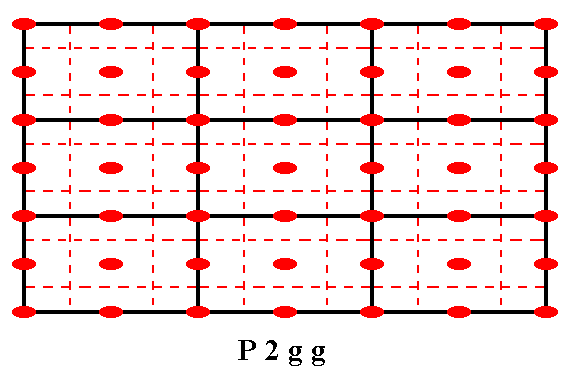Figure 2.  The total symmetry content of the Plane Group P2gg .
2-fold rotation axes perpendicular to the plane of the drawing are indicated by small solid red ellipses.
Glide lines are indicated by dashed red lines.
Mirror lines are absent.

```
```
The next Figure ilustrates one of the glide reflections present in the P2gg pattern.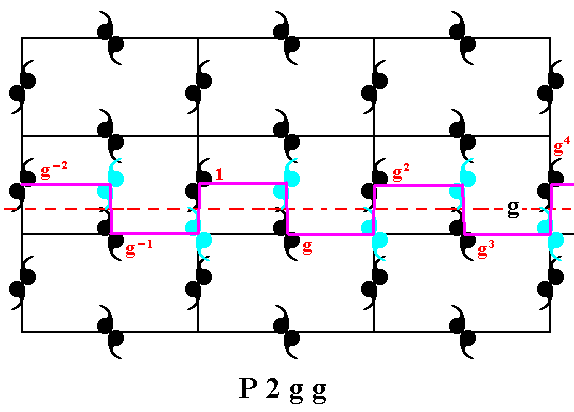Figure 3.  A glide reflection  g  in the P2gg pattern is indicated. Each (composed) motif consists of two motif units (commas), such that the composed motif possesses 2-fold rotational symmetry. One motif unit is chosen to figure as the initial motif unit from which the pattern is produced by (three) generators. Each motif unit represents a group element.

```
```Figure 4.  A  2-fold rotational symmetry is present at the point  R  (there are more such points). We will choose this rotation (1800 about R ) as one of the three generators of the group P2gg.

```
```
The next Figure indicates, in addittion to the one generator already chosen, the other two (chosen) generators. These three together can generate the whole pattern (and as such this repesents the generation of the group P2gg ).Figure 5.  The three chosen generators of the group P2gg :
The glide reflection
g ,  represented by the motif unit  g ,  the translation (vertical)  t ,  represented by the motif unit  t  and the half-turn  r  represented by the motif unit  r .

```
```
The next Figure shows how a number of group elements (represented by motif units) can be generated by means of the three chosen generators. Eventually all elements of the group can be generated.Figure 6.  Generation of the  P2gg  pattern by using the generators  g ,   t ,  r .
(For reasons of clarity of the drawing not all motif units are provided with their corresponding symbol).

```
```
As can be seen in the patterns of the above Figures, the "motifs" are not the units that are repeated over and over in the net. In fact they are just motif units (each in turn consisting of two ultimate motif units -- commas), and indeed two such units, taken together, units, that turn out to be symmetric with respect to each other (seen, when they are placed on the same level), are repeated.
In the next Figure we have considered the motif in the strict sense (motif s.str.) + its surroundings, making up the motif in the broad sense (motif s.l.). This latter motif is indeed repeated and tiles the 2-D plane completely.Figure 7.  The motif s.str. (black) and the motif s.l. (black + blue) in the pattern representing Plane Group P2gg .

```
```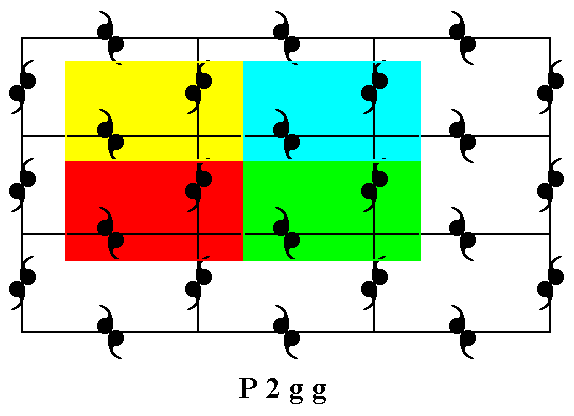Figure 8.  The motif s.l. is indeed repeated over and over again (four repeats are indicated), and tiles the entire 2-D plane.

```
```
If all translations in the pattern representing Plane Group P2gg are eliminated (by telescoping the structure into itself), then a figure will emerge that has 2mm symmetry. This shows that the Point Group associated with the Plane Group P2gg, i.e. the translation-free residue of that Plane Group, is 2mm, representing the Crystal Class based on this Plane Group. See Figure 9.Figure. 9.  The translation-free residue of the Plane Group P2gg is its Point Group. As can be seen, the figure (shape), representing this translation-free residue, has 2mm symmetry.

```
```
We will now extend the tiling (tesselation) of the plane by the motifs s.l.  as was depicted in Figure 8.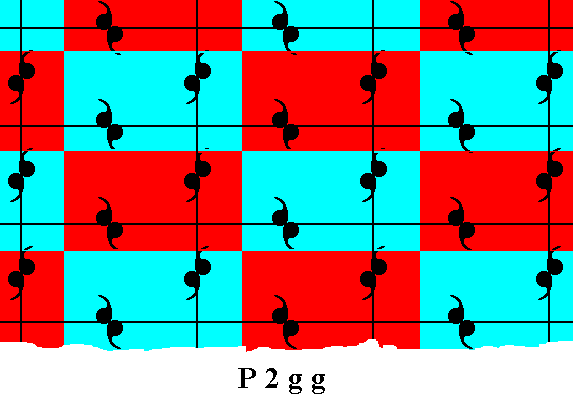Figure 10.  The motifs s.l.  (red and blue rectangles) of the  P2gg  pattern of Figure 1 tesselate the whole plane.

```
```
As can be seen in Figure 6, each motif unit s.str., i.e. each motif unit (comma) of a motif s.str., represents a group element.
We will now divide the motifs s.l. of Figure 10 in order to let the group elements be represented by the resulting subareas, such that the latter will tile the plane completely, as we have done in all previous cases. We will find out, however, that this is not possible with the present choice of motifs s.l., so we have to choose another type of area that tiles the plane completely and can be interpreted as a motif s.l., but at the same time is such that it can be divided into subareas that can represent group elements and also tile the plane completely. The next Figure depicts this alternative choice of motifs s.l., which satisfies the demands just stated.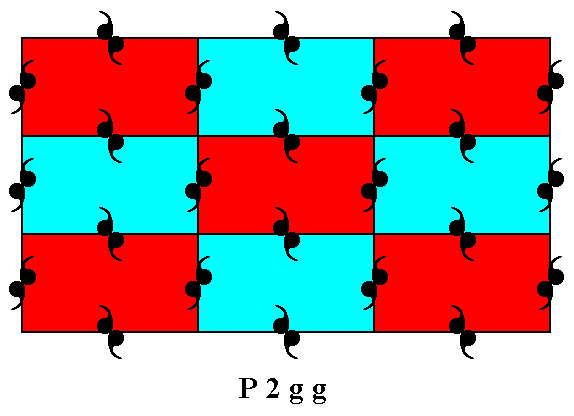Figure 11.  When we choose as motif s.l. the area enclosed by the lattice lines (See Figure 1), we get a motif s.l. that can be divided into areas correctly representing the group elements.

```
```
The next Figure gives such a motif s.l. as it is in itself, i.e. isolated from the rest of the pattern.Figure 12.  The newly chosen motif s.l.  isolated.

```
```
Although the alternative choice of motifs s.l. supports a division of them, such that the parts represent group elements correctly, these motifs (s.l.) are more abstract than those of the first choice, because now their boundaries go right through the motifs s.str.  Both choices of motif s.l. contain four ultimate motif units (commas) of motifs s.str.
The next Figure shows the division of the motifs s.l. as defined in Figures 11 and 12. And each of the parts of these motifs s.l., resulting from this division, can correctly represent group elements.Figure 13.  Division of the motifs s.l.  such that the rectangular (smaller) areas can represent group elements.

```
```
The next Figure indicates the initial group element and the three generators of the group, all in terms of corresponding areas of the pattern.Figure 14.  The initial group element  1  and the three generator group elements of the Plane Group  P2gg .
The generators are :
The glide reflection
g  (with respect to the glide line  g  indicated by the horizontal dashed line).
The half-turn
The vertical translation
t .

```
```
First we generate some group elements by subjecting the initial element 1 (repreatedly) to the glide reflection  g  :Figure 15.  Generation of the group elements  g-2 ,  g-1 ,   g2 ,  g3 ,  by applying the glide reflection -- the generator element  g -- (repeatedly) to the initial group element  1 .

```
```
The above action of the glide reflection  g  is highligted in the next Figure.Figure 16.  The action of the glide reflection  g .

```
```
The next Figure shows the generation of the group element  rg-1  from the element  g-1  by applying the half-turn about the point R .Figure 17.  Generation of the group element  rg-1  from the element  g-1  obtained earlier.

```
```
We now generate new group elements from the element  rg-1  by applying to it the translations  t-1  and  t-2 .Figure 18.  Generation of the group elements  t-1rg-1  and  t-2rg-1  from the element
rg-1  by applying to it the translations  t-1  and  t-2 .

```
```
By means of the half-turn  r  about the point R ,  which is a generator, we can generate yet a further group element :Figure 19.  Generation of the group element  rg  from the element  g  by appying to it the half-turn about the point  R .

```
```
Also from the element  g  we can generate new group elements,  tg  and  t2g ,  by applying the translations  t  and  t2 .Figure 20.  Generation of the group elements  tg  and  t2g .

```
```
By the half-turn about the point  R  we can generate yet another group element :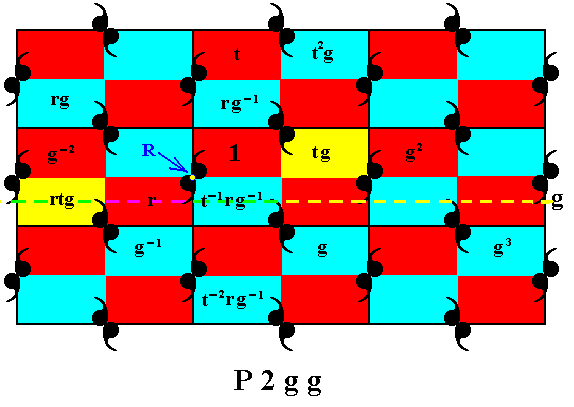Figure 21.  Generation of the group element  rtg .

```
```
Again by the half-turn about the point  R  we can generate yet another group element :Figure 21.  Generation of the group element  rt-1rg-1 ,  from the element  t-1rg-1 .

```
```
From the element  g-1  we can generate the element  t2g-1  :Figure 22.  Generation of the group element  t2g-1 .

```
```
From the element  r  we can generate the elements  tr  and  t-1r  :Figure 23.  Generation of the group elements  tr  and  t-1r .

```
```
From the group element  g2  we can generate two more group elements :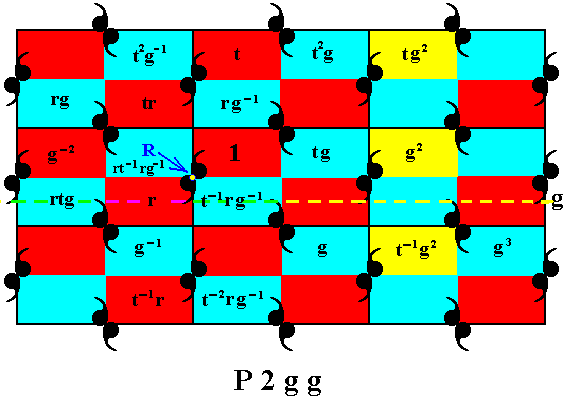Figure 24.  Generation of the group elements  tg2  and  t-1g2 .

```
```
From the element  rtg  we can generate yet another element :Figure 25.  Generation of the group element  t-1rtg .

```
```
From the group element  rt-1rg-1 we can generate more elements, by applying to it the glide reflections  g-1 ,  g ,  g4  (which all are implied by the generator  g ) :Figure 26.  Generation of the group elements  g-1rt-1rg-1 ,  grt-1rg-1 ,  g4rt-1rg-1 ,  by applying the glide reflections  g-1 ,  g ,  g4  to the element  rt-1rg-1 .

```
```
From the element  g-2  we can obtain the element  tg-2 ,  and from the element  g3  we can obtain the element  t2g3  :Figure 27.  Generation of the group elements  tg-2  and  t2g3 .

```
```
From the element  tg-2 the element  rtg-2  can be generated, by the half-turn about the point  R :Figure 28.  Generation of the group element  rtg-2 .

```
```
From the element  rtg-2  we can generate the elements  t2rtg-2  and  trtg-2  :Figure 29.  Generation of the group elements  t2rtg-2  and  trtg-2 .

```
```
From the element  rtg-2  we can generate the element  grtg-2  :Figure 30.  Generation of the group element  grtg-2 , by subjecting the element  rtg-2  to the glide reflection  g .

```
```
From the element  grtg-2  we can generate the elements  t-2grtg-2  and  t-1grtg-2  :Figure 31.  Generation of the group elements  t-2grtg-2  and  t-1grtg-2 .

```
```
From the element  t-2grtg-2  we can obtain the element  gt-2grtg-2 ,  by subjecting it to the glide reflection  g :Figure 32.  Generation of the group element  gt-2grtg-2 .

```
```
From the element  gt-2grtg-2  we can obtain the elements  t-1gt-2grtg-2  and  t-2gt-2grtg-2  by subjecting it to the translations  t-1  and  t-2 :Figure 33.  Generation of the group elements  t-1gt-2grtg-2  and  t-2gt-2grtg-2 .

```
```
Our 'final' result (the generation process goes on indefinitely of course) is depicted in the next Figure (it is the same as the previous Figure, but now with the auxiliary yellow colors and other auxiliary entities removed).Figure 34.  Final result of the generation of the group elements in the displayed part of the  P2gg  pattern.

```
```
The several group elements can of course be generated in different ways (with the same generators). We then get different, but wholly equivalent 'formulas' for the generated elements (the mentioned formulas, as given in the above Figures denote group elements as 'products' of other elements of the group. They express the successive execution of symmetry transformations). As an example of equivalent products, let's consider the group element denoted by  t2g3 ,  which we can find in the upper right corner of Figure 34. This group element was obtained from the element  g3 by applying to it the translation  t2 . But that same group element ( t2g3 ) can also be obtained from the element  g4rt-1rg-1 by subjecting it to the translation  t ,  resulting in the element  tg4rt-1rg-1. So we've found the algebraic relation (where "algebraic" stands for specifically that algebra that is defined by our group P2gg)

t2g3 = tg4rt-1rg-1

which just states that the two products are identical.
So there can be many alternative, but equivalent, denotations for group elements (in terms of generators).

As has been said, the generation process never comes to a conclusion, because the group is infinite. And this generation process is here again interpreted as a logical process which can, occasionally be expressed by the human mind, but which also as such subsists permanently and objectively in the Implicate Order, and, in the context of neoplatonic metaphysics, subsists in the hypostasis  S o u l  as a discursive thinking process, with  S o u l  as its subject, and (also) in the hypostasis  I n t e l l i g e n c e  as intuitive knowledge, where there is no distinction between (thinking) subject and (thought) object. In  S o u l  it is at the same time the formative field with respect to the internal symmetry of crystals, here explained by means of two-dimensional crystals, but meant to give some idea of all this with respect to real three-dimensional crystals.

In Plato-oriented philosophies there is a tendency of attributing to every  u n i v e r s a l  ( = more or less general concept) a transcendent existence, as an "Idea", in some higher sphere of existence, like  S o u l  (World-soul), or  I n t e l l i g e n c e.  Such an unlimited attribution could, however, lead to absurdities.
Here, however, it is held that only for concepts (as human thought structures) that are  i n t r i n s i c a l l y   o b j e c t i v e,  we can postulate a form of subsistence in some immaterial domain. Let's give some examples.
The concept "Crystal " stands for a collection of certain observable material objects. So in the sense of that to which that concept refers, the concept is objective. But,  a s   c o n c e p t   it is not objective. We cannot analyse the concept "Crystal " (or any other such concept), as to what it intrinsically is (apart from the fact that it is a thought pattern in our mind/brain), and what it entails, by itself, as concept. The only way to 'analyse' it is to investigate real crystals, but then we are not investigating a concept anymore.
Let's now take the concept "Group" (in the group theoretic sense).
This concept stands for a certain definable abstract structure (i.e. a particular algebraic structure). This structure is still of a conceptual nature, and it can be investigated. This is what group theorists do. So here we have an example of a concept (the concept "Group") that is  as concept  objective. And only such concepts (and the conceptual structures that can be built from them) we grant an independent subsistence in some immaterial sphere of being. In our neoplatonic context this sphere would be the hypostasis  S o u l  (World-soul), and the more exalted hypostasis  I n t e l l i g e n c e , and, finally, (such concepts subsisting in a totally integrated, ineffable and unknowable form in) the supreme hypostasis  T h e   O n e .

```

```
In the next document we will generate the Plane Group P2mg .

e-mail :```
```
To continue click HERE for further study of the totally dynamic and holistic nature of Reality.
```
```
back to homepage

****************